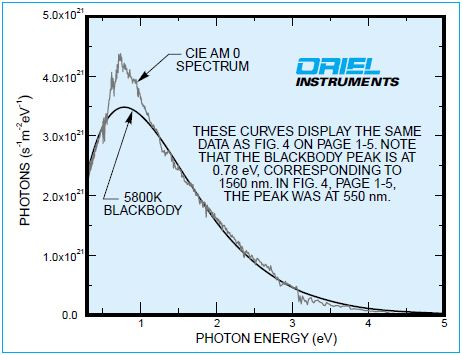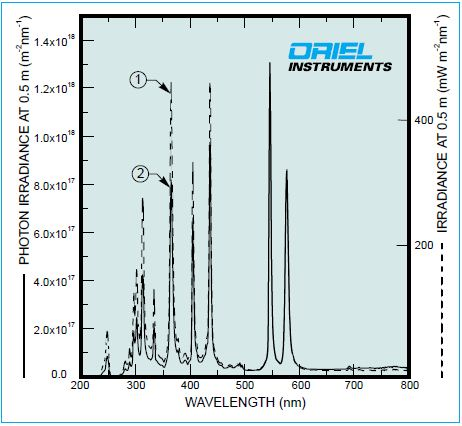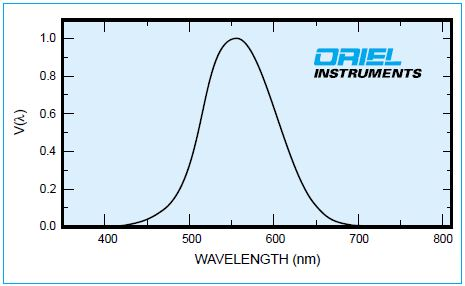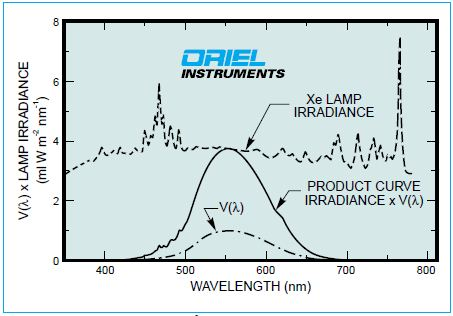# Introduction to Optical Radiation Terminology and Units

There are different systems of units for optical radiation. The article follows the internationally agreed CIE system. The CIE system fits well with the SI system of units.

## Radiometric, Photometric and Photon Quantities

Radiometric quantities are purely physical. How the human eye records optical radiation is more relevant when compared to the absolute physical values. This evaluation is described in photometric units and is limited to the small part of the spectrum called the visible. Photon quantities are essential for a number of physical processes. Table 1 lists radiometric, photometric and photon quantities.

Table 1. Commonly Used Radiometric, Photometric and Photon Quantities

Quantity Usual Symbol Units Quantity Usual Symbol Units Quantity Usual Symbol Units
Radiant Energy Qe J Luminous Energy Qv Im s Photon Energy Np *

Radiant Power or Flux φe W Luminous Flux φv Im Photon Flux Φp=dNp/dt s-1
Radiant Exitance or Emittance Me W m-2 Luminous Exitance or Emittance Mv Im m-2 Photon Exitance Mp s-1s-2
Radiant Intensity Ie W sr-1 Luminous Intensity Iv cd Photon Intensity Ip s-1sr-1
Radiance Le W sr-1 m-2 Luminance Lv cd m-2 Photon Radiance Lp s-1sr-1m-2

* Photon quantities are expressed in number of photons followed by the units, eg. photon flux (number of photons) s-1. The unit for photon energy is number of photons.

The subscripts e,v, and p designate radiometric, photometric, and photon quantities respectively. While working with only one type of quantity, they are normally omitted.

Symbols Key:

J: joule
lm: lumen
W: watts
s: second
m: meter
cd: candela
lx: lux, lumen m-2

Table 2. Some Units Still in Common Use

Units Equivalent Quantity
Talbot Im s Luminous Energy
Footcandle Im ft-2 Illuminance
Footlambert cd ft-2 Luminance
Lambert cd cm-2 Luminance

Sterance, aerance and pointance are used for supplementing or replacing the above terms.

• Sterance, means, related to the solid angle, so radiance may be described by radiant sterance.

## Spectral Distribution

Using spectral before the tabulated radiometric quantities implies consideration of the wavelength dependence of the quantity. The measurement wavelength should be given when a spectral radiometric value is quoted. The variation of spectral radiant exitance (M), or irradi-ance (E) with wavelength is often shown in a spectral distribution curve.

## Wavelength, Wavenumber, Frequency and Photon Energy

Wavelength is inversely proportional to the photon energy; shorter wavelength photons are more energetic photons. Wavenumber and frequency increase with photon energy.

The units of wavelength used are nanometers (nm) and micrometers(µm).

1 nm = 10-9 m = 10-3 μm
1 μm = 10-9 m = 1000 nm
1 Angstom unit (A) = 10-10 m = 10-1 nm

Figure 1 shows the solar spectrum and 5800K blackbody spectral distributions against energy (and wavenumber), in contrast with the familiar representation.

Table 2 below helps in converting from one spectral parameter to another. The conversions use the approximation 3 x 108 ms-1 for the speed of light. For precise work, the actual speed of light in the medium must be used. The speed in air is based on the wavelength, humidity and pressure, but the variance is only important for interferometry and high resolution spectroscopy.

Table 3. Spectral Parameter Conversion Factors

Wavelength Wavenumber* Frequency Photon Energy**
Symbol (Units) λ (nm) υ (cm-1) ν (Hz) Ep (eV)
Conversion Factors λ 107/ λ 3 x 1017 1,240/λ
107 υ 3 x 1010 υ 1.24 x 10-4υ
3 x 1017 3.33 x 10-11ν ν 4.1 x 10-15ν

1,240/Ep 8,056 x Ep 2.42 x 1014Ep Ep
Conversion Examples 200
500
1000
5 x 104
2 x 104
104
1.5 x 1015
6 x 1014
3 x 1014
6.20
2.48
1.24

* The S.I. unit is the m-1. Most users, primarily individuals working in infrared analysis, adhere to the cm-1.

** Photon energy is usually expressed in electron volts to relate to chemical bond strengths.The units are also more convenient than photon energy expressed in joules as the energy of a 500 nm photon is 3.98 x 10-19 J = 2.48 eV

Similarly by dividing by the beam impact area, photon irradiance can be easily calculated.

Most radiometric quantities as well as irradiance have values that are defined at a point even though the units, mW m2 nm1, imply a large area. The spatial map of the irradiance is required in the full description. Often average values over a defined area are most useful. Peak levels can greatly exceed average values.Figure 1. Unconventional display of solar irradiance on the outer atmosphere and the spectral distribution of a 5800K blackbody with the same total radiant flux.

## Converting from Radiometric to Photon Quantities

It is important to express radiation in photon quantities when irradiation results are described in terms of cross section, number of molecules excited or for many detector and energy conversion systems, quantum efficiency.

It is easy to calculate the number of photons in a joule of monochromatic light of wavelength λ as the energy in each photon is given by E = hc/λ joules

Where:

h = Planck's constant (6.626 x 10-34 Js), c = Speed of light (2.998 x 108 m s-1) λ = Wavelength in m

Hence the number of photons per joule is

N = λ x 5.03 x 1015 where λ is in nm+ Since a watt is a joule per second, one Watt of monochromatic radiation at λ corresponds to N photons per second. The general expression is:

dN/dt = Pλ x λ x 5.03 x 1015

where Pλ is in watts, λ is in nm

Table 4. Photopic Response

Wavelength (nm) Photopic Luminous Efficiency V(λ) Wavelength (nm) Photopic Luminous Efficiency V(λ)
380 0.00004 580 0.870
390 0.00012 590 0.757
400 0.0004 600 0.631
410 0.0012 610 0.503
420 0.0040 620 0.381
430 0.0116 630 0.265
440 0.023 640 0.175
450 0.038 650 0.107
460 0.060 660 0.061
470 0.091 670 0.032
480 0.139 680 0.017
490 0.208 690 0.0082
500 0.323 700 0.0041
510 0.503 710 0.0021
520 0.710 720 0.00105
530 0.862 730 0.00052
540 0.954 740 0.00025
550 0.995 750 0.00012
555 1.000 760 0.00006
560 0.995 770 0.00003
570 0.952

The spectral distribution of the radiations must be known for conversions. Conversion from a radiometric quantity (in watts) to the corresponding photometric quantity (in lumens) simply requires multiplying the spectral distribution curve by the photopic response curve, integrating the product curve and multiplying the result by a conversion factor of 683.

Mathematically for a photometric quantity (PQ) and its matching radiometric quantity (SPQ).

PQ = 683 ∫ (SPQλ) • V(λ)dλ

Since V(λ) is zero except between 380 and 770 nm, simply sum the product values over small spectral intervals, Δλ:

PQ ≈ (Σn (SPQλn) • V(λn)) • Δλ

Where:

(SPQλn) = Average value of the spectral radiometric quantity in wavelength interval number "n"

For conversion from radiometric to photon quantities, you need to know the spectral distribution of the radiation. For irradiance you need to know the dependence of E on X. The curves will have different shapes as shown in Figure 2.Figure 2. The wavelength dependence of the irradiance produced by the 6283 200 W mercury lamp at 0.5 m. (1) shown conventionally in mW m-2 nm-1 and (2) as photon flux.Figure 3. The normalized response of the "standard" light adapted eye.

## Conversion from Radiometric to Photometric Values

It is possible to convert from radiometric to matching photometric quantity.The photometric measure depends on how the source appears to the human eye.

Mathematically for a photometric quantity (PQ) and its matching radiometric quantity (SPQ).

PQ = 683 ∫ (SPQλ) • V(λ)dλ

Since Vλ is zero except between 380 and 770 nm, you only need to integrate over this range. Most computations simply sum the product values over small spectral intervals, Δλ

PQ ≈ (Σn (SPQλn) • V(λn)) • Δλ

Where:

(SPQλn) = Average value of the spectral radiometric quantity in wavelength interval number “n”

The smaller the wavelength interval and the slower the variation in SPQλ, the higher is the accuracy.Figure 4. Lamp Irradiance, V (λ), and product curve.

Figure 5 shows the irradiance curve multiplied by the V(λ) curve. The unit of the product curve that describes the radiation is the IW, or light watt, a hybrid unit bridging the transition between radiometry and photometry. The integral of the product curve is 396 mIWm-2, where a IW is the unit of the product curve.

Table 5. Light Watt Values

Wavelength Range (nm) Estimated Average Irradiance (mW m-2 nm-1) V(λ) Product of cols 1 & 2 x 50 nm (mIW m-2 )
380 - 430 3.6 0.0029 0.5
430 - 480 4.1 0.06 12
480 - 530 3.6 0.46 83
530 - 580 3.7 0.94 174
580 - 630 3.6 0.57 103
630 - 680 3.4 0.11 19
680 - 730 3.6 0.0055 1.0
730 - 780 3.8 0.0002 0.038

To get from IW to lumens requires multiplying by 683, so the illuminance is:

396x683mlumens m-2= 270lumensm-2 (or 270 lux)

Since there are 10.764 ft2 in a m2, the illuminance in foot candles (lumens ft-2) is 270/10.8 = 25.1 foot candles.

## Conclusion

The article offers an overview of the different units of optical radiation and conversion from one system to another.This information has been sourced, reviewed and adapted from materials provided by Oriel Instruments.

## Citations

• APA

Oriel Instruments. (2019, July 25). Introduction to Optical Radiation Terminology and Units. AZoOptics. Retrieved on October 22, 2020 from https://www.azooptics.com/Article.aspx?ArticleID=827.

• MLA

Oriel Instruments. "Introduction to Optical Radiation Terminology and Units". AZoOptics. 22 October 2020. <https://www.azooptics.com/Article.aspx?ArticleID=827>.

• Chicago

Oriel Instruments. "Introduction to Optical Radiation Terminology and Units". AZoOptics. https://www.azooptics.com/Article.aspx?ArticleID=827. (accessed October 22, 2020).

• Harvard

Oriel Instruments. 2019. Introduction to Optical Radiation Terminology and Units. AZoOptics, viewed 22 October 2020, https://www.azooptics.com/Article.aspx?ArticleID=827.